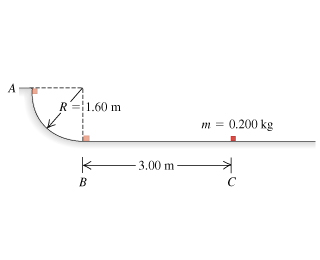# Problem: In a truck-loading station at a post office, a small 0.200-kg package is released from rest at point exttip{A}{A} on a track that is one-quarter of a circle with radius 1.60 m (the figure ). The size of the package is much less than 1.60 m, so the package can be treated as a particle. It slides down the track and reaches point exttip{B}{B} with a speed of 4.60 m/s . From point exttip{B}{B}, it slides on a level surface a distance of 3.00 m to point C, where it comes to rest.What is the coefficient of kinetic friction on the horizontal surface?How much work is done on the package by friction as it slides down the circular arc from exttip{A}{A} to exttip{B}{B}?

###### FREE Expert Solution
96% (209 ratings)
###### Problem Details

In a truck-loading station at a post office, a small 0.200-kg package is released from rest at point on a track that is one-quarter of a circle with radius 1.60 m (the figure). The size of the package is much less than 1.60 m, so the package can be treated as a particle. It slides down the track and reaches point with a speed of 4.60 m/s . From point , it slides on a level surface a distance of 3.00 m to point C, where it comes to rest.

What is the coefficient of kinetic friction on the horizontal surface?

How much work is done on the package by friction as it slides down the circular arc from to ?